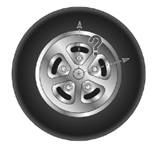Chapter 6.1, Problem 28EElementary Geometry For College St...

7th Edition
Alexander + 2 others
ISBN: 9781337614085

Solutions

Chapter
SectionElementary Geometry For College St...

7th Edition
Alexander + 2 others
ISBN: 9781337614085
Textbook Problem

A wheel has equally spaced lug bolts. What is the measure of the central angle determined by two consecutive lug bolts if there are a) 5 bolts? b) 6 bolts?To determine

a)

To calculate:

The measure of central angle determined by two consecutive lug bolts if there are 5 bolts.

Explanation

Given:

A wheel has equally spaced 5 lug bolts.

Postulate used:

Central angle postulate:

In a circle, the degree measure of a central angle is equal to the degree measure of its intercepted arc.

Congruent arcs:

In a circle or congruent circles, congruent arcs are arcs with equal measures.

Theorem:

In a circle or congruent circles, congruent minor arcs have congruent central angles.

Calculation:

Consider five bolts that are equally spaced on a wheel.

The wheel resembles the shape of a circle.

Thus the arcs formed by five bolts on the wheel are congruent arcs.

Hence if we consider the central angles for each arc, they will be congruent because the arcs are congruent...

To determine

b)

To calculate:

The measure of central angle determined by two consecutive lug bolts if there are 6 bolts.

Still sussing out bartleby?

Check out a sample textbook solution.

See a sample solution

The Solution to Your Study Problems

Bartleby provides explanations to thousands of textbook problems written by our experts, many with advanced degrees!

Get Started

Evaluate the integral. sin2sin6d

Calculus (MindTap Course List)

43. Find the slope of the line tangent to the graph of

Mathematical Applications for the Management, Life, and Social Sciences

True or False: The function f is continuous at (0, 0), where

Study Guide for Stewart's Multivariable Calculus, 8th

f(x) = (x2 3)2 has points of inflection at x = _____. a) 0,3,3 b) 1, 1 c) 0, 1, 1 d) 3,3

Study Guide for Stewart's Single Variable Calculus: Early Transcendentals, 8th

In Exercise 13-22, perform the indicated operations. 23

Finite Mathematics for the Managerial, Life, and Social Sciences